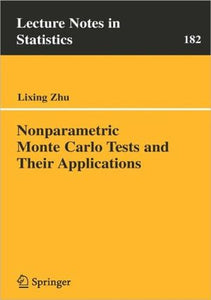Nonparametric Monte Carlo Tests And Their Applications (lecture Notes In Statistics)

Free shipping (Guaranteed delivery 3-5 business days for In-Stock products)
Out of stock

ISBN details

• ISBN 10: 0387250387
• ISBN 13: 9780387250380

Overview

A fundamental issue in statistical analysis is testing the fit of a particular probability model to a set of observed data. Monte Carlo approximation to the null distribution of the test provides a convenient and powerful means of testing model fit. Nonparametric Monte Carlo Tests and Their Applications proposes a new Monte Carlo-based methodology to construct this type of approximation when the model is semistructured. When there are no nuisance parameters to be estimated, the nonparametric Monte Carlo test can exactly maintain the significance level, and when nuisance parameters exist, this method can allow the test to asymptotically maintain the level.

The author addresses both applied and theoretical aspects of nonparametric Monte Carlo tests. The new methodology has been used for model checking in many fields of statistics, such as multivariate distribution theory, parametric and semiparametric regression models, multivariate regression models, varying-coefficient models with longitudinal data, heteroscedasticity, and homogeneity of covariance matrices. This book will be of interest to both practitioners and researchers investigating goodness-of-fit tests and resampling approximations.

Every chapter of the book includes algorithms, simulations, and theoretical deductions. The prerequisites for a full appreciation of the book are a modest knowledge of mathematical statistics and limit theorems in probability/empirical process theory. The less mathematically sophisticated reader will find Chapters 1, 2 and 6 to be a comprehensible introduction on how and where the new method can apply and the rest of the book to be a valuable reference for Monte Carlo test approximation and goodness-of-fit tests.

Lixing Zhu is Associate Professor of Statistics at the University of Hong Kong. He is a winner of the Humboldt Research Award at Alexander-von Humboldt Foundation of Germany and an elected Fellow of the Institute of Mathematical Statistics.
From the reviews:

"These lecture notes discuss several topics in goodness-of-fit testing, a classical area in statistical analysis. … The mathematical part contains detailed proofs of the theoretical results. Simulation studies illustrate the quality of the Monte Carlo approximation. … this book constitutes a recommendable contribution to an active area of current research." Winfried Stute for Mathematical Reviews, Issue 2006

"...Overall, this is an interesting book, which gives a nice introduction to this new and specific field of resampling methods." Dongsheng Tu for Biometrics, September 2006

Other Details

• Publisher: Springer New York
• Language: Eng
• Edition: 2005
• Dimensions: 8.27 (w) x 5.83 (h) x 0.45 (d)
• Pages: 196
• Date Published: 2005
• Authors: Lixing Zhu